# AP Board 3rd Class Maths Solutions 4th Lesson Subtraction

Andhra Pradesh AP Board 3rd Class Maths Solutions 4th Lesson Subtraction Textbook Exercise Questions and Answers.

## AP State Syllabus 3rd Class Maths Solutions Chapter 4 Subtraction

Textbook Page No. 42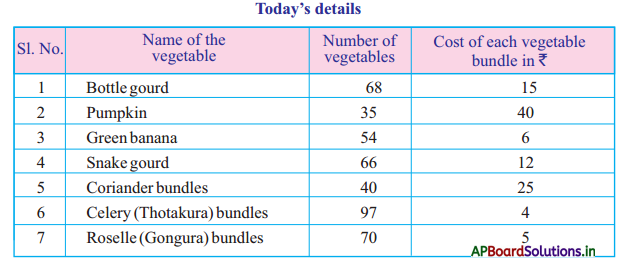Now, answer the following questions from the above information.

Question 1.
What leafy vegetables that Mariyamma yielded ?
Corriander, celery, Rosella

Question 2.
Which leafy vegetables bundle costs more today ?
Corriander bundlesQuestion 3.
How many more bottle gourds are harvested than pumpkin today ?
68 – 35 = 33

Question 4.
How many less green bananas are harvested than snake gourd ?
66 – 54 = 12

Question 5.
If you want one hundred bundles of Roselle, how many more bundles are required ?
100 – 70 = 30 bundles are required

Textbook Page No. 44

Do the following.

a)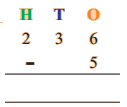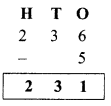b)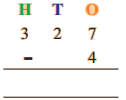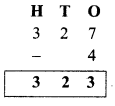c)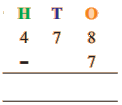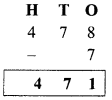d)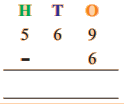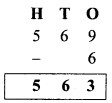Textbook Page No. 45

Do these:
Do the following.

Question 1.
a)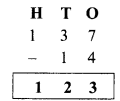b)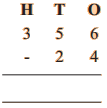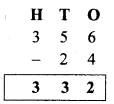c)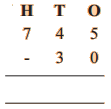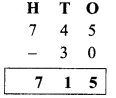d)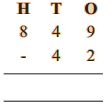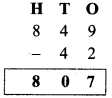Question 2.
There are 247 birds sitting on a tree. Out of them 42 flew away. How many birds are there on the tree ?
Number of birds sitting on the tree = 247
Number of birds flew away = 42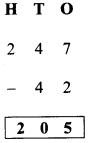Number of birds remained on the tree = 205

Question 3.
The cost of a shirt is ₹ 385. The price is reduced by ₹ 35 for a festive season. What is the price of the shirt after reduction in price ?
The cost of a shirt = 385
Price reduced = 35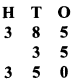Actual price of the shirt = 350

Textbook Page No. 47

Exercise 1

1. Do the following:

a)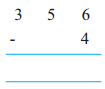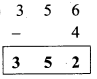b)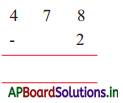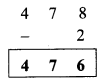c)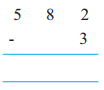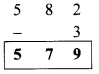d)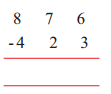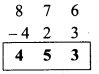e)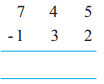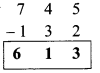f)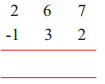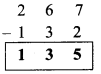g)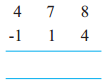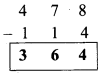h)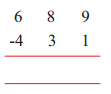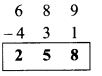2. Answer the following oral questions.

a) What is the difference between 300 and 200 ?
The difference between 300 and 200 is 100.

b) What will we get, if we subtract 125 from 175 ?
50 will get when we subtract 125 from 175.

Question 3.
How many rupees are needed to make a sum of ₹ 425 to ₹ 679?
Required money to get ₹ 679 from ₹425 is = 679 – 425 = ₹ 254

Question 4.
There are 385 students in a school. Mid Day Meal agency has 142 eggs with them. How many more eggs are required to distribute each one an egg?
Number of students in a school = 385
Number of eggs at agency = 142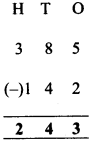Required more eggs are = 243

Textbook Page No. 49

Do these:

Question 1.
Do the following.

a)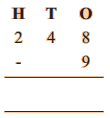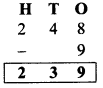b)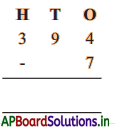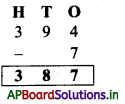c)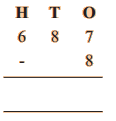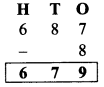Textbook Page No. 51

Do the following:

Question 1.
a)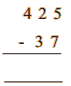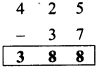b)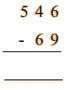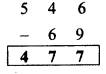c)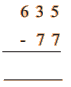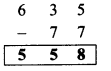Question 2.
Rajayya has 342 sheep. He sold 65 sheep. How many sheep are there now?
Number of sheep at Rajayya = 342
Number of sheep sold = (-) 65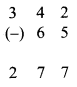Remaining sheep at Rajayya = 277

Textbook Page No. 53

Exercise – 2

1. Do the following.

a)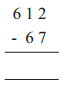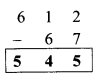b)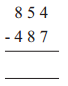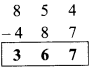c)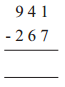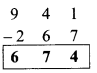d)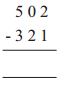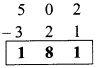e)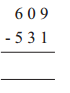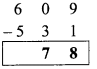f)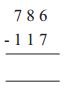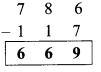g)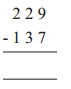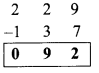h)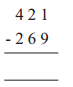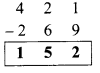i)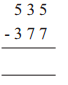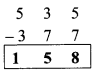j)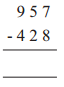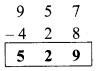Question 2.
Balu has done some subtraction sums given below. Verify and correct the mistakes.
a)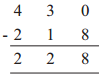228 is wrong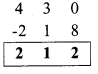b)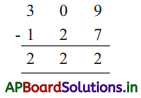222 is wrong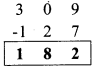c)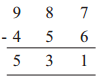531 is correct

d)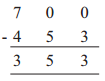353 is wrong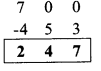Question 3.
If 235 hens are sold from 520 hens in a poultry, how many hens are remaining ?
Number of hens in poultry = 520
Number of hens sold = 235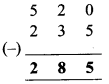Remaining hens = 2 8 5

Textbook Page No. 54

Question 4.
Match the following. One is done for you.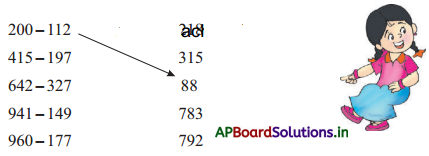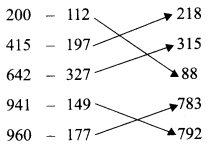Question 5.
There are 432 students in a school. Among them, 245 are girls. How many boys are there?
Number of students in School = 432
Number of girls = 245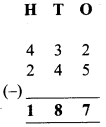Number of boys = 187

Question 6.
The cost of a pair of Chappal is ₹ 250. If Shiva has ₹ 195 with him, how much more amount is required to buy the Chappals ?
The cost of a pair of chappal is = ₹ 250
Money at Shiva = ₹ 195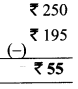Required money to Shiva ₹ 55

Question 7.
Naresh has ₹ 500/- with him. Which
1) Shirt = ₹ 200
2) Pant = ₹ 300
3) Shoes = ₹ 400
4) Bag = ₹ 300
5) Ball = ₹ 200
6) Water bottle = ₹ 100
7) Bat = ₹ 400
Amount at Naresh = ₹ 500
Naresh buys following items with amount at him.

1. Shirt – 1 and pant – 1
2. Shoes – 1 and water bottle – 1
3. Bag – 1 and Ball – 1
4. Bat – 1 and water bottle – 1

Do these:

Question 1.
Age of Vahida is 39 years. Her daughter, Karima’s age is 21 years less than her age. What is the age of Karima ?
Age of vahida = 39 years
Karima’s age is 21 years less than her mother Vahida.
Age of Karima = 39 – 21 = 18 years.

Question 2.
There are 650 eggs in a school. In which, 579 eggs are used to serve in lunch. How many eggs remain ?
Number of eggs in a school = 650
Number of eggs used = 579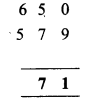Remaining eggs = 71Question 3.
I have some money. If you give ₹ 200 to me, my money will become ₹ 780. How much money do I have already?
Let the money at me = ₹ x
Received money from you = ₹ 200
Total my money becomes = ₹ 780
At first money at me ₹ = 780 – 200
= ₹ 580

Textbook Page No. 55

Do these:

Estimate the difference and circle to the nearer one.

a)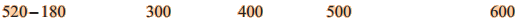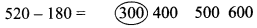b)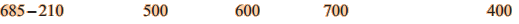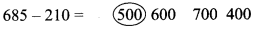Multiple Choice Questions

Question 1.
The difference between 685 and 210 ( )
A) 400
B) 465
C) 475
D) 485
C) 475

Question 2.
Who was called “Human Computer” ( )
A) Ramanujan
C) RammohanQuestion 3.
Sohan went to a rice store to buy a rice bag costs ₹ 850. He gave ₹900. Then amount he got back ? ( )
A) ₹ 100
B) ₹ 50
C) ₹ 75
D) ₹ 25
B) ₹ 50

Question 4.
The difference of375 – 215 is nearer to ( )
A) 100
B) 50
C) 200
D) 250